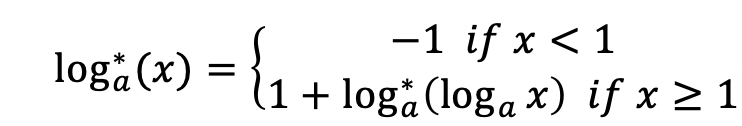×

# 计蒜客

1. 题库
2. super_log
3. 问答
• 16.16%
• 131072K

In Complexity theory, some functions are nearly \$O(1)\$, but it is greater then \$O(1)\$. For example, the complexity of a typical disjoint set is \$O(nα(n))\$. Here \$α(n)\$ is Inverse Ackermann Function, which growth speed is very slow. So in practical application, we often assume \$α(n) \le 4\$.

However \$O(α(n))\$ is greater than \$O(1)\$, that means if \$n\$ is large enough, \$α(n)\$ can greater than any constant value.

Now your task is let another slowly function \$log*\$ \$x\$ reach a constant value \$b\$. Here \$log*\$ is iterated logarithm function, it means “the number of times the logarithm function iteratively applied on \$x\$ before the result is less than logarithm base \$a\$”.

Formally, consider a iterated logarithm function \$log_{a}^* \$Find the minimum positive integer argument \$x\$, let \$log_{a}^* (x) \ge b\$. The answer may be very large, so just print the result \$x\$ after mod \$m\$.

### Input

The first line of the input is a single integer \$T(T\le 300)\$ indicating the number of test cases.

Each of the following lines contains \$3\$ integers \$a\$ , \$b\$ and \$m\$.

\$1 \le a \le 1000000\$

\$0 \le b \le 1000000\$

\$1 \le m \le 1000000\$

Note that if a==1, we consider the minimum number x is 1.

### Output

For each test case, output \$x\$ mod \$m\$ in a single line.

### Hint

In the \$4-th\$ query, \$a=3\$ and \$b=2\$. Then \$log_{3}^* (27) = 1+ log_{3}^* (3) = 2 + log_{3}^* (1)=3+(-1)=2 \ge b\$, so the output is \$27\$ mod \$16 = 11\$.

```5
2 0 3
3 1 2
3 1 100
3 2 16
5 3 233```

```1
1
3
11
223```

• main.c